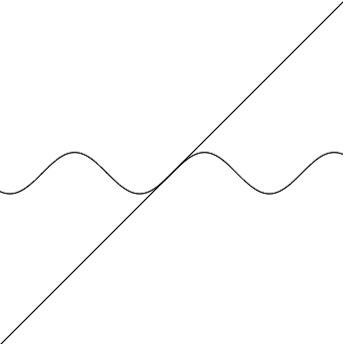Calculus

# Calculus Warmups: Level 3 ChallengesIs $f(x)= x + \sin x$ an injective function?

$\large\sum _{ j=0 }^{ \infty }{ \sum _{ i=0 }^{ \infty }{ \frac { { 2 }^{ -(i+j) } }{ i+j+1 } } } = \ ?$

$\large \lim _{xy\to 1}\left(\frac{\ln\:x}{\ln\:y}+\frac{\ln\:y}{\ln\:x}\right)= \ ?$

$\large 2 \times \lim_{h\to0} \dfrac{\int_1^{1+h} x^x \, dx - \int_{1-h}^1 x^x \, dx }{h^2} =\, ?$

Some people claim that $0 ^ 0 = 1$. What is

$\Large \lim_{ x \rightarrow 0^+ } x ^{^ { \frac{ 1}{\ln x} }}?$

×